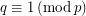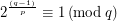# Special Primes

 Importance: Low ✭
 Author(s): George BALAN
 Subject: Number Theory
 Keywords:
 Posted by: maththebalans on: February 18th, 2011
Conjecture   Letbe a prime natural number. Find all primes, such that.

## Bibliography

* indicates original appearance(s) of problem.

### All primes are

All primes are p=(q-1)/(order of 2 mod q)

### paul newell

q divides 2^((q-1)/P))-1 iff p divides (q-1)/( Order of2 mod q )